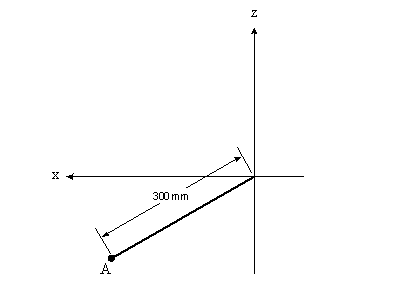# Statics cable length problem

sevag00

## Homework Statement

The window is held open by the cable AB. Determine the length of the cable and express the 30N acting at A along the cable as a Cartesian vector.
http://img802.imageshack.us/img802/9599/ru5y.jpg [Broken]

vector(F) = FuAB

## The Attempt at a Solution

Here's my solution
http://img833.imageshack.us/img833/6996/n7b0.png [Broken]

Last edited by a moderator:

## Answers and Replies

Homework Helper
Check the x and z coordinates of A.

ehild

Staff Emeritus
Homework Helper
Check the coordinates of B.

sevag00
Yeah the B coordinates should be B(0,150,250) but i don't know what's wrong with the A coordinates especially the z one.

Homework Helper
The plate is not horizontal, but inclined at 30°angle downward. 300 mm is the length of the edge.

ehild

sevag00
300mm is the length of the edge which is the x coordinate, just like 500 which is the y coordinate.

Mentor
300mm is the length of the edge which is the x coordinate, just like 500 which is the y coordinate.

Nope. Sketch the side view:EDIT: Added point A to the diagram.

#### Attachments

Last edited:
sevag00
But isn't the y of point A at the end of the window and not at 0?

Mentor
But isn't the y of point A at the end of the window and not at 0?

Yes. The sketch is looking along the y-axis (the y-axis is coming straight out of the page), so all y-coordinates will be seen as flat against the x-z plane. Point A is at the end of the heavy line, not at the origin.

sevag00
So in this case, what is the coordinate?

Mentor
So the y coordinate will be 300, right?

How do you get that? I thought we were talking about the x and z coordinates for point A.

Mentor
So in this case, what is the coordinate?

I modified my diagram to show the location of point A (I thought it was obvious, but it never hurts to clarify). What x and z coordinates would you assign to point A?

sevag00
So z=-300sin30 x=300cos30 and y=500.

sevag00
How do you get that? I thought we were talking about the x and z coordinates for point A.

No. That was stupid. I edited my post.

Mentor
So z=-300sin30 x=300cos30 and y=500.

Yes, that looks better.

sevag00
Thanks for the help.